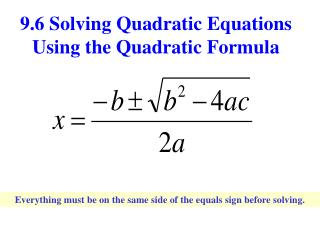DownloadDownload Presentation9.6 Solving Quadratic Equations Using the Quadratic Formula

# 9.6 Solving Quadratic Equations Using the Quadratic Formula

Download Presentation## 9.6 Solving Quadratic Equations Using the Quadratic Formula

- - - - - - - - - - - - - - - - - - - - - - - - - - - E N D - - - - - - - - - - - - - - - - - - - - - - - - - - -
##### Presentation Transcript

1. 9.6 Solving Quadratic Equations Using the Quadratic Formula Everything must be on the same side of the equals sign before solving.

2. You must memorize this formula! Time to sing along!!

3. Pop Goes the Weasel X equals neg-a-tive b Plus or minus the square root B squared minus 4 A C ALL over 2 A

4. The Flintstones Negative B Plus or mi-nus Square root B squared minus 4 A C A – L – L, over 2 A That’s the quadratic form-u-lee.

5. 1st 2nd Ex: 1 Solve x2+ 9x +14 = 0 a = 1 b = 9 c =14

6. Ex: 2 Solve x2 +x = 1 a = 1 b = 1 c = -1

7. Ex: 3 Solve 2x2 – 7x +5 = 0 a = 2 b = -7 c = 5

8. Ex: 4 Solve x2 + 6x – 3 = 0 a = 1 b = 6 c = -3